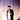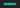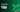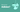Navigate back to the homepageIshan Sharma
June 5th, 2020 · 11 min readRecently, a few weeks ago, I was browsing on the internet and searching for some C++ related concepts and got prompted with a link to the foobar challenge by google. This challenge acts as a recruiting tool for google. This Google foobar site allows access to questions only for people with a invite.In this post, I’ll write about the challenge, the problems that were posed for me in the challenge and my approach.

The Google Foobar challenge consisted of 5 Levels.
Level 1 consisted of a single question.           [48 hours/question]
Level 2 consisted of two questions.                [72 hours/question]
Level 3 consisted of three questions.             [96 hours/question]
Level 4 consisted of two questions.                [15 days/question]
Lastly, Level 5 consisted of one question.     [24 days/question]

## Level 1

• ### Problem a : ‘The cake is not a lie!’

Commander Lambda has had an incredibly successful week: she completed the first test run of her LAMBCHOP doomsday device, she captured six key members of the Bunny Rebellion, and she beat her personal high score in Tetris. To celebrate, she’s ordered cake for everyone - even the lowliest of minions! But competition among minions is fierce, and if you don’t cut exactly equal slices of cake for everyone, you’ll get in big trouble.

The cake is round, and decorated with M&Ms in a circle around the edge. But while the rest of the cake is uniform, the M&Ms are not: there are multiple colors, and every minion must get exactly the same sequence of M&Ms. Commander Lambda hates waste and will not tolerate any leftovers, so you also want to make sure you can serve the entire cake.

To help you best cut the cake, you have turned the sequence of colors of the M&Ms on the cake into a string: each possible letter (between a and z) corresponds to a unique color, and the sequence of M&Ms is given clockwise (the decorations form a circle around the outer edge of the cake).

Write a function called solution(s) that, given a non-empty string less than 200 characters in length describing the sequence of M&Ms, returns the maximum number of equal parts that can be cut from the cake without leaving any leftovers.

• ##### Languages
• To provide a Python solution, edit solution.py
• To provide a Java solution, edit Solution.java
• ##### Test Cases

Your code should pass the following test cases. Note that it may also be run against hidden test cases not shown here.

• Python Cases

`.css-1chxjt6{position:absolute;right:22px;top:24px;padding:8px 12px 7px;border-radius:5px;color:#6f7177;-webkit-transition:background 0.3s ease;transition:background 0.3s ease;}.css-1chxjt6:hover{background:rgba(255,255,255,0.07);}.css-1chxjt6[data-a11y="true"]:focus::after{content:"";position:absolute;left:-2%;top:-2%;width:104%;height:104%;border:2px solid var(--theme-ui-colors-accent,#6166DC);border-radius:5px;background:rgba(255,255,255,0.01);}@media (max-width:45.9375em){.css-1chxjt6{display:none;}}1Input:2solution.solution("abcabcabcabc")3Output:445Input:6solution.solution("abccbaabccba")7Output: 2`
• Java Cases

`1Solution.solution("abcabcabcabc")2Output: 434Input:5Solution.solution("abccbaabccba")6Output: 2`
• ## Approach

To solve this, The first thing I did was to visualize the problem, so as to get a better understanding. I just imagined watching a round cake from top view and assigned few letters (a,b,c,d) to different sections of it in a repeating pattern.

Now that we have visualized it. We can easily infer that, basically all we have to do is to look for repeating sub-strings in the string.

Of course, We need to keep important things in mind before we begin actually writing the code, The function would need to return 0 if the cake could not be cut without leftovers or if the string was empty. We need to identify possible M&M patterns from the input string. The pattern surely could not be longer than half the length of the string. We need to check to see if there were remainders when chopping the string with the possible patterns and discard those results.

`1def substring_check(length, sub_str):2    for i in range(0, length-1):3        if(sub_str[i] == sub_str[i+1]):4            if(i == length-2):5                return length6            else:7                continue8        else:9            return 11011def solution(s):12    parts = 1   #Initially, Let's just assume that cake can be divided into just one piece1314    for i in range(1, len(s)):15        sub_str = []16        if(len(s) % i == 0):17            for j in range(0, len(s), i):18                sub_str.append(s[j:j+i])19            parts = substring_check(len(sub_str), sub_str)20            if(parts != 1):21                return parts22    return parts`

and Voila! just like that we have completed the first Level of Foobar Challenge!

## Level 2

• ### Problem a : ‘Lovely Lucky Lambs’

Being a henchman isn’t all drudgery. Occasionally, when Commander Lambda is feeling generous, she’ll hand out Lucky LAMBs (Lambda’s All-purpose Money Bucks). Henchmen can use Lucky LAMBs to buy things like a second pair of socks, a pillow for their bunks, or even a third daily meal!

However, actually passing out LAMBs isn’t easy. Each henchman squad has a strict seniority ranking which must be respected - or else the henchmen will revolt and you’ll all get demoted back to minions again!

There are 4 key rules which you must follow in order to avoid a revolt:

1. The most junior henchman (with the least seniority) gets exactly 1 LAMB. (There will always be at least 1 henchman on a team.)
2. A henchman will revolt if the person who ranks immediately above them gets more than double the number of LAMBs they do.
3. A henchman will revolt if the amount of LAMBs given to their next two subordinates combined is more than the number of LAMBs they get. (Note that the two most junior henchmen won’t have two subordinates, so this rule doesn’t apply to them. The 2nd most junior henchman would require at least as many LAMBs as the most junior henchman.)
4. You can always find more henchmen to pay - the Commander has plenty of employees. If there are enough LAMBs left over such that another henchman could be added as the most senior while obeying the other rules, you must always add and pay that henchman.

Note that you may not be able to hand out all the LAMBs. A single LAMB cannot be subdivided. That is, all henchmen must get a positive integer number of LAMBs.

Write a function called solution(total_lambs), where total_lambs is the integer number of LAMBs in the handout you are trying to divide. It should return an integer which represents the difference between the minimum and maximum number of henchmen who can share the LAMBs (that is, being as generous as possible to those you pay and as stingy as possible, respectively) while still obeying all of the above rules to avoid a revolt. For instance, if you had 10 LAMBs and were as generous as possible, you could only pay 3 henchmen (1, 2, and 4 LAMBs, in order of ascending seniority), whereas if you were as stingy as possible, you could pay 4 henchmen (1, 1, 2, and 3 LAMBs). Therefore, solution(10) should return 4-3 = 1.

To keep things interesting, Commander Lambda varies the sizes of the Lucky LAMB payouts. You can expect total_lambs to always be a positive integer less than 1 billion (10 ^ 9).

• ##### Languages
• To provide a Python solution, edit solution.py
• To provide a Java solution, edit Solution.java
• ##### Test Cases

Your code should pass the following test cases. Note that it may also be run against hidden test cases not shown here.

• Python Cases

`1Input  : solution.solution(143)2Output : 334Input  : solution.solution(10)5Output : 1`
• Java Cases

`1Input  : Solution.solution(143)2Output : 334Input  : Solution.solution(10)5Output : 1`
• ## Approach

At first glance, this question seems a bit tricky. Basically, they are asking the difference between no. of Fibonacci numbers whose sum is less than total_lambs and no. of powers of 2 whose sum is less than total_lambs (* a catch in the rule number 4).

Once we grasp the hold of the question, coming up with the solution is pretty easy.

`1def solution(total_lambs):2    # Your code here3    maxlist=[]4    i=05    henchmen=06    while i<= total_lambs:7        currentvalue=2**i8        maxlist.append(currentvalue)9        henchmen=henchmen + currentvalue10        if henchmen > total_lambs:11            break12        i=i+11314    lambs=[1,1]15    fibhenchmen=216    y=217    while y<= total_lambs:18        value=lambs[y-1] + lambs[y-2]19        lambs.append(value)20        fibhenchmen=fibhenchmen + int(lambs[y])21        if fibhenchmen > total_lambs:22            break23        y=y+12425    answer = len(lambs) - len(maxlist)2627    return abs(answer)`
• ### Problem b : ‘Hey! I already did that.’

Commander Lambda uses an automated algorithm to assign minions randomly to tasks, in order to keep her minions on their toes. But you’ve noticed a flaw in the algorithm - it eventually loops back on itself, so that instead of assigning new minions as it iterates, it gets stuck in a cycle of values so that the same minions end up doing the same tasks over and over again. You think proving this to Commander Lambda will help you make a case for your next promotion.

You have worked out that the algorithm has the following process:

1. Start with a random minion ID n, which is a nonnegative integer of length k in base b
2. Define x and y as integers of length k. x has the digits of n in descending order, and y has the digits of n in ascending order
3. Define z = x - y. Add leading zeros to z to maintain length k if necessary
4. Assign n = z to get the next minion ID, and go back to step 2

For example, given minion ID n = 1211, k = 4, b = 10, then x = 2111, y = 1112 and z = 2111 - 1112 = 0999. Then the next minion ID will be n = 0999 and the algorithm iterates again: x = 9990, y = 0999 and z = 9990 - 0999 = 8991, and so on.

Depending on the values of n, k (derived from n), and b, at some point the algorithm reaches a cycle, such as by reaching a constant value. For example, starting with n = 210022, k = 6, b = 3, the algorithm will reach the cycle of values [210111, 122221, 102212] and it will stay in this cycle no matter how many times it continues iterating. Starting with n = 1211, the routine will reach the integer 6174, and since 7641 - 1467 is 6174, it will stay as that value no matter how many times it iterates.

Given a minion ID as a string n representing a nonnegative integer of length k in base b, where 2 <= k <= 9 and 2 <= b <= 10, write a function solution(n, b) which returns the length of the ending cycle of the algorithm above starting with n. For instance, in the example above, solution(210022, 3) would return 3, since iterating on 102212 would return to 210111 when done in base 3. If the algorithm reaches a constant, such as 0, then the length is 1.

• ##### Languages
• To provide a Python solution, edit solution.py
• To provide a Java solution, edit Solution.java
• ##### Test Cases

Your code should pass the following test cases. Note that it may also be run against hidden test cases not shown here.

• Python Cases

`1Input  : solution.solution('210022', 3)2Output : 33Input  : solution.solution('1211', 10)4Output : 1`
• Java Cases

`1Input  : Solution.solution('210022', 3)2Output : 3`

Input : Solution.solution(‘1211’, 10) Output : 1

`1`
• ## Approach

This seemed quite difficult to grasp when I first read the question, I just got puzzled with all that ’mumble-jumble’. So, I read the problem again, for a few times & then just started coding my way to achieve the desired results, as stated by the algorithm process above.

`Please note that the functions 'dTob' & 'bTod' actually represent decimal to binary and binary to decimal conversions respectively.`

`1def dTob(d, b):2    digits = []3    while d > 0:4        digits.insert(0, str(d % b))5        d  = d / b6    return ''.join(digits)78def bTod(b, c):9  n = 010  for d in str(b):11    n = c * n + int(d)12  return n1314def negative(x, y, b):15  if b==10:16    return int(x) - int(y)1718  dx=bTod(x,b)19  dy=bTod(y,b)20  dz=dx-dy21  return dTob(dz, b)2223def solution(n, b):24    arr=[]25    while True:26        i = "".join(sorted(str(n), reverse=True))27        j = "".join(sorted(str(n)))28        k = negative(i,j,b)2930        k2 = len(str(k))31        i2 = len(str(i))3233        if (k2) != i2:34            k = k * pow(10 ,(i2-k2))3536        for index, item in enumerate(arr):37          if item == k:38            return index + 139        arr = [k] + arr40        n = k`

With the above problems out of the way, we can actually proceed to much more interesting challenges.

## Level 3

• ### Problem a : ‘Fuel Injection Perfection’

Commander Lambda has asked for your help to refine the automatic quantum antimatter fuel injection system for her LAMBCHOP doomsday device. It’s a great chance for you to get a closer look at the LAMBCHOP - and maybe sneak in a bit of sabotage while you’re at it - so you took the job gladly.

Quantum antimatter fuel comes in small pellets, which is convenient since the many moving parts of the LAMBCHOP each need to be fed fuel one pellet at a time. However, minions dump pellets in bulk into the fuel intake. You need to figure out the most efficient way to sort and shift the pellets down to a single pellet at a time.

The fuel control mechanisms have three operations:

2. Remove one fuel pellet
3. Divide the entire group of fuel pellets by 2 (due to the destructive energy released when a quantum antimatter pellet is cut in half, the safety controls will only allow this to happen if there is an even number of pellets)

Write a function called solution(n) which takes a positive integer as a string and returns the minimum number of operations needed to transform the number of pellets to 1. The fuel intake control panel can only display a number up to 309 digits long, so there won’t ever be more pellets than you can express in that many digits.

For example:
solution(4) returns 2: 4 -> 2 -> 1
solution(15) returns 5: 15 -> 16 -> 8 -> 4 -> 2 -> 1

• ##### Languages
• To provide a Python solution, edit solution.py
• To provide a Java solution, edit Solution.java
• ##### Test Cases

Your code should pass the following test cases. Note that it may also be run against hidden test cases not shown here.

• Python Cases

`1Input  : solution.solution('15')2Output : 534Input  : solution.solution('4')5Output : 2`
• Java Cases

`1Input  : Solution.solution('15')2Output : 534Input  : Solution.solution('4')5Output : 2`
• ## Approach

I believe this problem statement is quite easy to grasp and doesn’t need much explanation, basically we have an input number and we have to change that number to 1, by performing any of the three operations defined above.

We have to return the minimum possible number of operations required to achieve the same.

`1def solution(n):23  n=int(n)4  steps=056  while(n!=1):7      # IF EVEN, I CAN DIVIDE IT BY 28      if(n%2==0):9          n=n/210      # ELSE IF NOT EVEN, I NEED TO EITHER ADD OR DELETE ONE11      # FUEL PALLETE, WHICHEVER EASIER12      elif((n==3) or (n%4==1)):13          n-=114      else:15          n+=11617      #INCREASE THE NO. OF STEPS18      steps=steps+11920  return steps`
• ### Problem b : ‘Bomb, Baby!’

You’re so close to destroying the LAMBCHOP doomsday device you can taste it! But in order to do so, you need to deploy special self-replicating bombs designed for you by the brightest scientists on Bunny Planet. There are two types: Mach bombs (M) and Facula bombs (F). The bombs, once released into the LAMBCHOP’s inner workings, will automatically deploy to all the strategic points you’ve identified and destroy them at the same time.

But there’s a few catches. First, the bombs self-replicate via one of two distinct processes: Every Mach bomb retrieves a sync unit from a Facula bomb; for every Mach bomb, a Facula bomb is created; Every Facula bomb spontaneously creates a Mach bomb.

For example, if you had 3 Mach bombs and 2 Facula bombs, they could either produce 3 Mach bombs and 5 Facula bombs, or 5 Mach bombs and 2 Facula bombs. The replication process can be changed each cycle.

Second, you need to ensure that you have exactly the right number of Mach and Facula bombs to destroy the LAMBCHOP device. Too few, and the device might survive. Too many, and you might overload the mass capacitors and create a singularity at the heart of the space station - not good!

And finally, you were only able to smuggle one of each type of bomb - one Mach, one Facula - aboard the ship when you arrived, so that’s all you have to start with. (Thus it may be impossible to deploy the bombs to destroy the LAMBCHOP, but that’s not going to stop you from trying!)

You need to know how many replication cycles (generations) it will take to generate the correct amount of bombs to destroy the LAMBCHOP. Write a function solution(M, F) where M and F are the number of Mach and Facula bombs needed. Return the fewest number of generations (as a string) that need to pass before you’ll have the exact number of bombs necessary to destroy the LAMBCHOP, or the string “impossible” if this can’t be done! M and F will be string representations of positive integers no larger than 10^50. For example, if M = “2” and F = “1”, one generation would need to pass, so the solution would be “1”. However, if M = “2” and F = “4”, it would not be possible.

• ##### Languages
• To provide a Python solution, edit solution.py
• To provide a Java solution, edit Solution.java
• ##### Test Cases

Your code should pass the following test cases. Note that it may also be run against hidden test cases not shown here.

• Python Cases

`1Input  : solution.solution('2', '1')2Output : 134Input  : solution.solution('4','7')5Output : 4`
• Java Cases

`1Input  : Solution.solution('2', '1')2Output : 134Input  : Solution.solution('4','7')5Output : 4`
• ## Approach

From the first look, what I can infer is that I basically have to find the number of subtractions that are required to find the Greatest Common Divisor of M & F.

`1def solution(x, y):23    x, y = int(x), int(y)4    # 'x' is for Mach Bombs5    # 'y' is for Facula Bombs67    cycles = 08    # Counts the number of replication cycles910    while (x != 1 and y != 1):1112        # If number of Mach bombs and Facula bombs are same13        # Then, It is impossible to replicate to the desired14        # Total number of bombs1516        if x % y == 0:17            return "impossible"1819        else:20            # cycles = cycles + 121            # ABOVE ASSIGNMENT, CAUSES TWO TEST CASES TO FAIL22            # FOR EXAMPLE FOR 7/3 : 2 CYCLES ARE CONSUMED23            cycles = cycles+int(max(x, y)/min(x, y))2425            x, y = max(x, y) % min(x, y), min(x, y)2627    return str(cycles+max(x, y)-1)`
• ### Problem c : ‘Queue To Do’

You’re almost ready to make your move to destroy the LAMBCHOP doomsday device, but the security checkpoints that guard the underlying systems of the LAMBCHOP are going to be a problem. You were able to take one down without tripping any alarms, which is great! Except that as Commander Lambda’s assistant, you’ve learned that the checkpoints are about to come under automated review, which means that your sabotage will be discovered and your cover blown - unless you can trick the automated review system.

To trick the system, you’ll need to write a program to return the same security checksum that the guards would have after they would have checked all the workers through. Fortunately, Commander Lambda’s desire for efficiency won’t allow for hours-long lines, so the checkpoint guards have found ways to quicken the pass-through rate. Instead of checking each and every worker coming through, the guards instead go over everyone in line while noting their security IDs, then allow the line to fill back up. Once they’ve done that they go over the line again, this time leaving off the last worker. They continue doing this, leaving off one more worker from the line each time but recording the security IDs of those they do check, until they skip the entire line, at which point they XOR the IDs of all the workers they noted into a checksum and then take off for lunch. Fortunately, the workers’ orderly nature causes them to always line up in numerical order without any gaps.

For example, if the first worker in line has ID 0 and the security checkpoint line holds three workers, the process would look like this:

•  0 1 2 / 3 4 / 5 6 / 7 8

where the guards’ XOR (^) checksum is 0^1^2^3^4^6 == 2.

Likewise, if the first worker has ID 17 and the checkpoint holds four workers, the process would look like:

•  17 18 19 20 / 21 22 23 / 24 25 26 / 27 28 29 / 30 31 32
which produces the checksum 17^18^19^20^21^22^23^25^26^29 == 14.

All worker IDs (including the first worker) are between 0 and 2000000000 inclusive, and the checkpoint line will always be at least 1 worker long.

With this information, write a function solution(start, length) that will cover for the missing security checkpoint by outputting the same checksum the guards would normally submit before lunch. You have just enough time to find out the ID of the first worker to be checked (start) and the length of the line (length) before the automatic review occurs, so your program must generate the proper checksum with just those two values.

• ##### Languages
• To provide a Python solution, edit solution.py
• To provide a Java solution, edit Solution.java
• ##### Test Cases

Your code should pass the following test cases. Note that it may also be run against hidden test cases not shown here.

• Python Cases

`1Input  : solution.solution(0, 3)2Output : 234Input  : solution.solution(17, 4)5Output : 14`
• Java Cases

`1Input  : Solution.solution(0, 3)2Output : 234Input  : Solution.solution(17, 4)5Output : 14`
• ## Approach

From the first look, what I can infer is that I basically have to find the number of subtractions that are required to find the Greatest Common Divisor of M & F.

`1def solution(x, y):23    x, y = int(x), int(y)4    # 'x' is for Mach Bombs5    # 'y' is for Facula Bombs67    cycles = 08    # Counts the number of replication cycles910    while (x != 1 and y != 1):1112        # If number of Mach bombs and Facula bombs are same13        # Then, It is impossible to replicate to the desired14        # Total number of bombs1516        if x % y == 0:17            return "impossible"1819        else:20            # cycles = cycles + 121            # ABOVE ASSIGNMENT, CAUSES TWO TEST CASES TO FAIL22            # FOR EXAMPLE FOR 7/3 : 2 CYCLES ARE CONSUMED23            cycles = cycles+int(max(x, y)/min(x, y))2425            x, y = max(x, y) % min(x, y), min(x, y)2627    return str(cycles+max(x, y)-1)`

and with that out of the way, we have finally now succesfully completed Level 3 of the Foobar Challenge.

Let’s get some rest and we’ll move to much more interesting & ‘mind-boggling’ challenges, afterwards.

### Join my mailing list and get notified about new blogs

Be the first to receive my latest content with the ability to opt-out at anytime. Of course, I promise that I won't spam your inbox or share your email with any third parties.

### More articles from Ishan Sharma### Growing Up

Reflections on the elusive concept of growing up and the moments in life that have prompted me to question my age and maturity

September 4th, 2023 · 4 min read### Why do I love Adidas?

Discover why adidas captures my heart with its perfect blend of comfort, simplicity, and sustainable innovation

July 2nd, 2023 · 4 min read